JMSLTM Numerical Library 7.2.0
com.imsl.stat

## Class KaplanMeierEstimates

• All Implemented Interfaces:
Serializable, Cloneable

```public class KaplanMeierEstimates
extends Object
implements Serializable, Cloneable```
Computes Kaplan-Meier (or product-limit) estimates of survival probabilities for a sample of failure times that possibly contain right consoring.

Class `KaplanMeierEstimates` computes Kaplan-Meier (or product-limit) estimates of survival probabilities for a sample of failure times that can be right censored or exact times. A survival probability S(t) is defined as 1 - F(t), where F(t) is the cumulative distribution function of the failure times t. Greenwood's estimate of the standard errors of the survival probability estimates are also computed. (See Kalbfleisch and Prentice, 1980, pages 13 and 14.)

Let (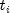,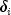), for i = 1,..., n denote the failure censoring times and the censoring codes for the n observations in a single sample. Here,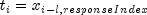is a failure time ifis 0, where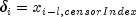. Also,is a right censoring time ifis 1. Rows in `x` containing values other than 0 or 1 forare ignored. Let the number of observations in the sample that have not failed by time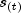be denoted by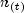, whereis an ordered (from smallest to largest) listing of the distinct failure times (censoring times are omitted). Then the Kaplan-Meier estimate of the survival probabilities is a step function, which in the interval from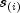to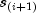(including the lower endpoint) is given by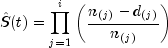where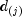denotes the number of failures occurring at time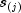, and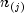is the number of observations that have not failed prior to.

Note that one row of `x` may correspond to more than one failed (or censored) observation when the frequency option is in effect (see `setFrequencyColumn`). The Kaplan-Meier estimate of the survival probability prior to time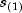is 1.0, while the Kaplan-Meier estimate of the survival probability after the last failure time is not defined.

Greenwood's estimate of the variance of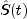in the interval fromtois given as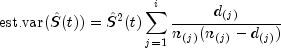`KaplanMeierEstimates` computes the single sample estimates of the survival probabilities for all samples of data included in `x` during a single call. This is accomplished through the stratum column of `x`, which if present, must contain a distinct code for each sample of observations (see `setStratumColumn`). If a stratum column is not specified, there is no grouping , and all observations are assumed to come from the same sample.

When failures and right-censored observations are tied and the data are to be sorted by `KaplanMeierEstimates` (`setSorted(true)` is not used), `KaplanMeierEstimates` assumes that the time of censoring for the tied-censored observations is immediately after the tied failure (within the same sample). When `setSorted(true)` is used, the data are assumed to be sorted from smallest to largest according to the response time column of `x` within each stratum (see `setResponseColumn`). Furthermore, a small increment of time is assumed (theoretically) to elapse between the failed and censored observations that are tied (in the same sample). Thus, when `setSorted(true)` is used, the user must sort all of the data in `x` from smallest to largest according to the response time column (and the stratum column, if set). By appropriate sorting of the observations, the user can handle censored and failed observations that are tied in any manner desired.

Example , Serialized Form
• ### Constructor Summary

Constructors
Constructor and Description
`KaplanMeierEstimates(double[][] x)`
Constructor for `KaplanMeierEstimates`.
• ### Method Summary

Methods
Modifier and Type Method and Description
`int` `getCensorColumn()`
Returns the column index of `x` containing the optional censoring code for each observation.
`int` `getFrequencyColumn()`
Returns the column index of `x` containing the frequency of response for each observation.
`int` `getGroupTotal(double groupValue)`
Returns the total number in the group for the specified group value.
`double` `getLogLikelihood(double groupValue)`
Returns the Kaplan-Meier log-likelihood of the group with the specified group value.
`int[]` `getNumberAtRisk()`
Returns the number of individuals at risk at each failure point.
`int[]` `getNumberOfFailures()`
Returns the number of failures which occurred at each failure point.
`int` `getNumberOfRowsMissing()`
Returns the number of rows of data in `x` that contain missing values in one or more specific columns of `x`.
`int` `getResponseColumn()`
Returns the column index of `x` containing the response time for each observation.
`double[]` `getStandardErrors()`
Returns Greenwood's estimated standard errors.
`int` `getStratumColumn()`
Returns the column index of `x` containing the stratum number for each observation.
`double[]` `getSurvivalProbabilities()`
Returns the estimated survival probabilities.
`int` `getTotalNumberOfFailures(double groupValue)`
Returns the total number failing in the group for the specified group value.
`void` `setCensorColumn(int censorIndex)`
Sets the column index of `x` containing the optional censoring code for each observation.
`void` `setFrequencyColumn(int frequencyIndex)`
Sets the column index of `x` containing the frequency of response for each observation.
`void` `setResponseColumn(int responseIndex)`
Sets the column index of `x` containing the response time for each observation.
`void` `setSorted(boolean isSorted)`
Sets the `boolean` to indicate that the column of response times in `x` are already sorted.
`void` `setStratumColumn(int stratumIndex)`
Sets the column index of `x` containing the stratum number for each observation.
• ### Methods inherited from class java.lang.Object

`clone, equals, finalize, getClass, hashCode, notify, notifyAll, toString, wait, wait, wait`
• ### Constructor Detail

• #### KaplanMeierEstimates

`public KaplanMeierEstimates(double[][] x)`
Constructor for `KaplanMeierEstimates`.
Parameters:
`x` - a `double` matrix containing the data, including optional data. By default it is assumed the response times are in column 0.
• ### Method Detail

• #### getCensorColumn

`public int getCensorColumn()`
Returns the column index of `x` containing the optional censoring code for each observation.
Returns:
an `int` specifying the column index of `x` containing the optional censoring code for each observation.
• #### getFrequencyColumn

`public int getFrequencyColumn()`
Returns the column index of `x` containing the frequency of response for each observation.
Returns:
an `int` specifying the column index of `x` containing the frequency of response for each observation.
• #### getGroupTotal

`public int getGroupTotal(double groupValue)`
Returns the total number in the group for the specified group value.
Parameters:
`groupValue` - a `double` specifying the group value.
Returns:
an `int` representing the total number in the group which has value `groupValue`.
• #### getLogLikelihood

`public double getLogLikelihood(double groupValue)`
Returns the Kaplan-Meier log-likelihood of the group with the specified group value.

The Kaplan-Meier log-likelihood is computed as: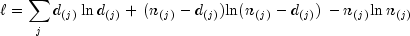where the sum is with respect to the distinct failure times.

Parameters:
`groupValue` - a `double` specifying the group value.
Returns:
a `double` representing the Kaplan-Meier log-likelihood of the group which has value `groupValue`.
• #### getNumberAtRisk

`public int[] getNumberAtRisk()`
Returns the number of individuals at risk at each failure point.
Returns:
an `int` array containing the number of individuals at risk at each failure point.
• #### getNumberOfFailures

`public int[] getNumberOfFailures()`
Returns the number of failures which occurred at each failure point.
Returns:
an `int` array containing the number of failures which occurred at each failure point.
• #### getNumberOfRowsMissing

`public int getNumberOfRowsMissing()`
Returns the number of rows of data in `x` that contain missing values in one or more specific columns of `x`.
Returns:
an `int` scalar representing the number of rows of data in `x` that contain missing values in one or more specific columns of `x`.
• #### getResponseColumn

`public int getResponseColumn()`
Returns the column index of `x` containing the response time for each observation.
Returns:
an `int` specifying the column index of `x` containing the response time for each observation.
• #### getStandardErrors

`public double[] getStandardErrors()`
Returns Greenwood's estimated standard errors.
Returns:
a `double` array containing Greenwood's estimate of the standard errors for the survival probabilities.
• #### getStratumColumn

`public int getStratumColumn()`
Returns the column index of `x` containing the stratum number for each observation.
Returns:
an `int` specifying the column index of `x` containing the stratum number for each observation.
• #### getSurvivalProbabilities

`public double[] getSurvivalProbabilities()`
Returns the estimated survival probabilities.
Returns:
a `double` array containing the estimated survival probabilities.
• #### getTotalNumberOfFailures

`public int getTotalNumberOfFailures(double groupValue)`
Returns the total number failing in the group for the specified group value.
Parameters:
`groupValue` - a `double` specifying the group value.
Returns:
an `int` representing the total number failing in the group which has value `groupValue`.
• #### setCensorColumn

`public void setCensorColumn(int censorIndex)`
Sets the column index of `x` containing the optional censoring code for each observation.
Parameters:
`censorIndex` - an `int` specifying the column index of `x` containing the optional censoring code for each observation. If `x[i][censorIndex]` equals 0, the failure time `x[i][responseIndex]` is treated as an exact time of failure. Otherwise, it is treated as right-censored time. Default: It is assumed that there is no censor code column in `x`. All observations are assumed to be exact failure times.
• #### setFrequencyColumn

`public void setFrequencyColumn(int frequencyIndex)`
Sets the column index of `x` containing the frequency of response for each observation.
Parameters:
`frequencyIndex` - an `int` specifying the column index of `x` containing the frequency of response for each observation. Default: It is assumed that there is no frequency response column recorded in `x`. Each observation in the data array is assumed to be for a single failure.
• #### setResponseColumn

`public void setResponseColumn(int responseIndex)`
Sets the column index of `x` containing the response time for each observation.
Parameters:
`responseIndex` - an `int` specifying the column index of `x` containing the response time for each observation. The interpretation of these times as either right-consored or exact failure times depends on the setting of the censor codes in the censor code column. See method `setCensorColumn`. Default: `responseIndex = 0`.
• #### setSorted

`public void setSorted(boolean isSorted)`
Sets the `boolean` to indicate that the column of response times in `x` are already sorted.
Parameters:
`isSorted` - a `boolean` indicating whether or not column `responseIndex` of `x` is already sorted. `isSorted` equal to `true` indicates that column `responseIndex` of `x` is already sorted. Otherwise, a detached sort is performed prior to analysis. If sorting is performed, all censored individuals are assumed to follow tied failures. Default: It is assumed that column `responseIndex` of `x` is not sorted, so a detached sort is performed.
• #### setStratumColumn

`public void setStratumColumn(int stratumIndex)`
Sets the column index of `x` containing the stratum number for each observation.
Parameters:
`stratumIndex` - an `int` specifying the column index of `x` containing the stratum number for each observation. Column `stratumIndex` of `x` contains a unique value for each stratum in the data. Kaplan-Meier estimates are computed within each stratum. Default: It is assumed that there is no stratum number column recorded `x`. The data is assumed to come from one statum.
JMSLTM Numerical Library 7.2.0